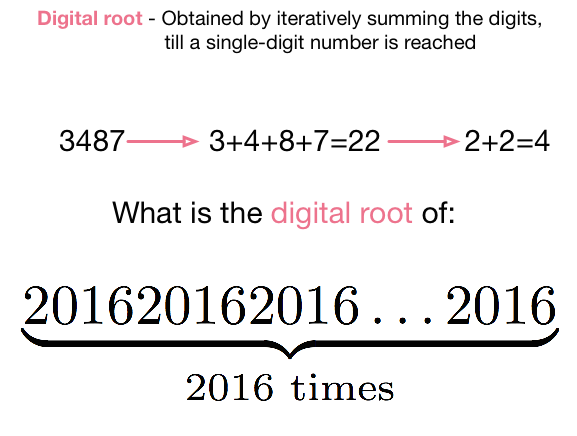# 2016 Digital RootFor each number $n$, we define the Digital Root of $n$ in the following manner: Take the sum of the digits to obtain a new number. Repeat this process until the result is a single digit.

Find the digital root of $\underset { 2016\text{ times} }{ \underbrace { 2016\ldots2016 } }$.

As an explicit example, for $n=3487$, $3487\quad \rightarrow \quad 3+4+8+7=22\quad \rightarrow \quad 2+2=4 \; .$ Therefore, the digital root of 3487 is 4.

Clarification: In the number above, 2016 appears 2016 times in its decimal representation.

×